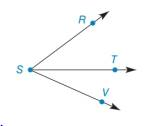Chapter 1.4, Problem 23E### Elementary Geometry for College St...

6th Edition
Daniel C. Alexander + 1 other
ISBN: 9781285195698

#### Solutions

Chapter
Section### Elementary Geometry for College St...

6th Edition
Daniel C. Alexander + 1 other
ISBN: 9781285195698
Textbook Problem
1 views

#Exercises 16 − 24 Given: S T →   bisects  ∠ R S V m ∠ R S T = x + y m ∠ T S V = 2 x − 2 y m ∠ R S V = 64 ° Find: x     and  y

To determine

To find:

The value of x and y for given information.

Explanation

Given:

STbisects RSVmRST=x+ymTSV=2x2ymRSV=64°

The given figure is,

Definition:

If a point say M lies in the interior of PQR, then mPQM+mRQM=mPQR.

Calculation:

Consider the figure,

T is the interior point of the angle RSV.

mRST=x+ymTSV=2x2ymRSV=64°

Use above mentioned angle addition postulate to solve for the value of x.

mRST+mTSV=mRSVx+y+2x2y=64

Simplify above equation,

3xy=64(1)

It is given that ST is the bisector of RSV

### Still sussing out bartleby?

Check out a sample textbook solution.

See a sample solution

#### The Solution to Your Study Problems

Bartleby provides explanations to thousands of textbook problems written by our experts, many with advanced degrees!

Get Started

#### Find more solutions based on key concepts